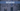# Remove Duplicates from Sorted Array - Leet Code Solution

August 26, 2020## Problem Statement

Given a sorted array nums, remove the duplicates in-place such that each element appear only once and return the new length.

Note:

1. We have to return the new length
2. And, modify the array also

Constraint
Do not allocate extra space for another array, you must do this by modifying the input array in-place with O(1) extra memory.

### Examples

``````# Example 1
Given nums = [1,1,2],
Output = 2

# Example 2
Given nums = [0,0,1,1,1,2,2,3,3,4],
Output = 5``````

## Solution (Using an extra variable)

First think out loud about the problem.

• Its a sorted array,
• And, which means a number which is repeating would occur later after another number.

### Code

Lets look at the code

``````public int removeDuplicates(int[] nums) {
if (nums == null || nums.length == 0) {
return 0;
}
int num = nums;
int j=1;

for (int i=1; i<nums.length; i++) {
if (num != nums[i]) {
num = nums[i];

nums[j] = nums[i];
j ++;
}
}

return j;
}``````

Steps

• We can begin with first index value, save it in a variable.
• We can keep another variable for storing our index `j`, which will point to index upto which our array is unique.
• This index variable will increment after finding a unique value only.
• And, we need to copy that unique value to our array as well.

### Results

``````Runtime: 0 ms, faster than 100.00% of Java online submissions for Remove Duplicates from Sorted Array.
Memory Usage: 41.1 MB, less than 87.80% of Java online submissions for Remove Duplicates from Sorted Array.``````

### Code without extra variable

``````public int removeDuplicates2(int[] nums) {
if (nums == null || nums.length == 0) {
return 0;
}
int j=1;
for (int i=1; i<nums.length; i++) {
if (nums[j-1]!= nums[i]) {
nums[j]= nums[i];

j ++;
}
}

return j;
}``````